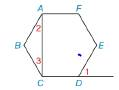Chapter 2.5, Problem 30EElementary Geometry For College St...

7th Edition
Alexander + 2 others
ISBN: 9781337614085

Solutions

Chapter
SectionElementary Geometry For College St...

7th Edition
Alexander + 2 others
ISBN: 9781337614085
Textbook Problem

Given: Regular hexagon ABCDEF with diagonal A ¯ C ¯ and exterior ∠ 1 . Prove: m ∠ 2+m ∠ 3 = m ∠ 1To determine

To prove:

m2+m3=m1

Explanation

Given:

The following figure shows the given diagram.

Figure (1)

EDC is supplementary to 1 as shown in given figure.

Calculation:

The completed proof is shown in the following table.

 Proof Statements Reasons 1. The ∠EDC is supplementary to ∠1 1. Given 2.m∠1+m∠EDC=180° 2. The sum of measures of two supplementary angles is 180° 3.m∠B+m∠2+m∠3=180° 3. The sum of measures of four angles in a quadrilateral is 180°. 4. Regular hexagon ABCDEF 4. Given 5.m∠B=m∠EDC 5. The measures of interior angles in a regular hexagon will be same. 6.m∠2+m∠3=m∠1 6. Solve (2), (3), and (5)

Conclusion:

Thus, the completed proof is shown in the following table

Still sussing out bartleby?

Check out a sample textbook solution.

See a sample solution

The Solution to Your Study Problems

Bartleby provides explanations to thousands of textbook problems written by our experts, many with advanced degrees!

Get Started

Find more solutions based on key concepts